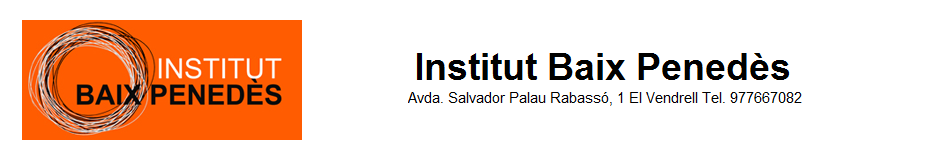# WIMS: WWW Interactive Multipurpose Server

interactive exercises, online calculators and plotters, mathematical recreation and games

Keywords: interactive mathematics, interactive math, server side interactivityDocuments Other languages: caesfrit

## Activities

Matrix multiplier, input two matrices and get their product (or other formula).
Wcalc, multi-purpose single-step calculator usable in popup mode.
Line choice, exercise : recognize a line by equation or by graph.
Quizz matrices, elementary questions on matrices.
OEF Matrices, collection of exercises on matrices.
OEF derivatives, collection of exercises on derivatives of functions of one variable.
OEF periodic table, exercises for memorising the periodic table of elements.
Parmsys, analyse a linear system with parameters, using Gauss elimination.
Popup calculator forms, you can search calculating forms or insert them into your own web pages.
OEF derivative, practising with differentiation.
OEF Determinant, collection of exercises on the determinant of a square matrix.
Derivative dialog, ask questions to get information in order to compute derivatives.
OEF linear systems, collection of exercises on linear systems.
Genspace, does a given set of vectors generate the whole vector space?.
OEF vectors 3D, collection of exercises on 3D vectors.
MatEq, asks to solve matrix equations.
Quizz determinant, elementary questions on the determinant of a matrix.
OEF vectors 2D, collection of exercises on 2D vectors.
In order to access WIMS services, you need a browser supporting forms. In order to test the browser you are using, please type the word wims here: and press Enter''.